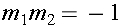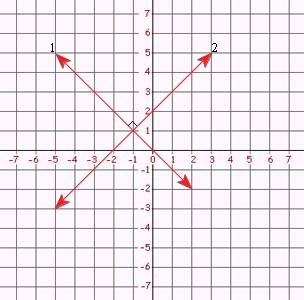Coordinate System (Parallel/Perpendicular/Intercepts)

Coordinate System

The coordinate system is a grid system, similar to a map. Its lines are formed by two axes that are drawn perpendicular to each other.  The horizontal axis is called the x-axis, and the vertical axis is called the y-axis.  The two intersecting axes form four quadrants, numbered I through IV.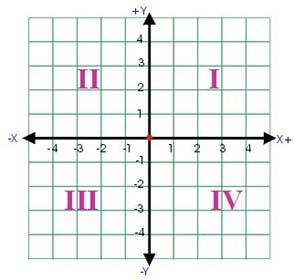The point of intersection (0, 0) is called the origin.  In an ordered pair, the x-coordinate is always listed first and the y-coordinate second.

Intercept

The x-intercept is the point at which a graph crosses the x-axis.  The y-intercept is the point at which the graph crosses the y-axis. To find the x-intercept of a line, substitute 0 for y into the equation and solve for x. To find the y-intercept of a line, substitute 0 for x into the equation and solve for y. You can use the intercepts to graph the line.

Example 1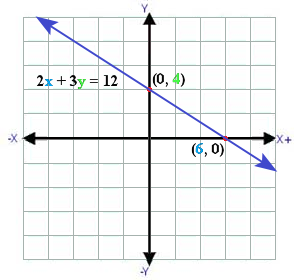The y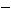intercept is 4

The xintercept is 6

• The graph of 2x + 3y = 12 crosses the x-axis at (6, 0), so its x-intercept is 6.
• The graph of 2x + 3y = 12 crosses the y-axis at (0, 4), so its y-intercept is 4.

Example 2

Find the intercepts of the graph

y = x5

Solution

To find the x-intercept let y = 0 and solve for x

y = x5
0 = x5
5 = x
10 = x
To find the y-intercept let x = 0 and solve for y
y = x5
y = (0)5
y = 05
y =5

The x-intercept is 10, and the y-intercept is -5.

The graph of the equation contains the points (10, 0) and (0,-5).  You could plot these two points and connect them to determine the graph of the line.

Compare the Slopes of Lines

Two lines in a plane are parallel if they do not intersect. Two lines in a plane are perpendicular if they intersect to form a right angle. The slope can be used to determine whether two different lines are parallel or perpendicular.

Consider two different non-vertical lines 1 and 2. Line 1 has a slope, m1, and line 2 has a slope, m2. The lines are parallel if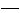and only ifthey have the same slope; that would make m1 = m2.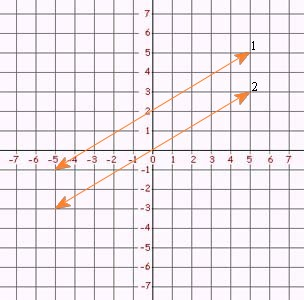The lines are perpendicular  if  their slopes are negative reciprocals of each other. Therefore, perpendicular lines have the following relationships between their slopes: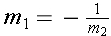or